Octave – Basics of Plotting Data

• Last Updated : 03 Nov, 2021

Octave has some in-built functions for visualizing the data. Few simple plots can give us a better way to understand our data. Whenever we perform a learning algorithm on an Octave environment, we can get a better sense of that algorithm and analyze it. Octave has lots of simple tools that we can use for a better understanding of our algorithm.
In this tutorial, we are going to learn how to plot data for better visualization and understanding it in the Octave environment.
Example 1 : Plotting a sine wave using the plot() and and sin() function:

MATLAB

 % var_x for the y-axisvar_x = [0:0.01:1]; % var_y for the y-axisvar_y = sin(4 * pi * var); % plotting the graphplot(var_x, var_y);

Output :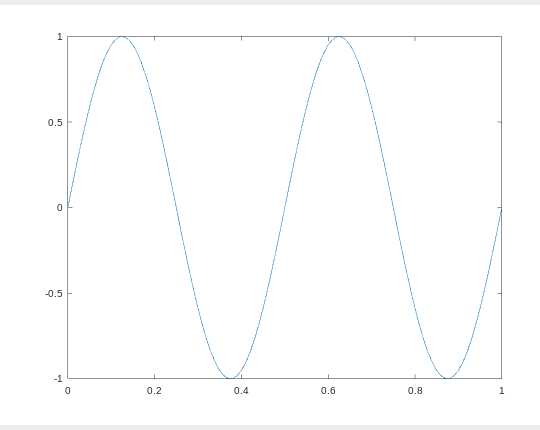Example 2 : Plotting a cosine wave using the plot() and and cos() function:

MATLAB

 % var_x for the y-axisvar_x = [0:0.01:1]; % var_y for the y-axisvar_y = cos(3 * pi * var); % plotting the graphplot(var_x, var_y);

Output :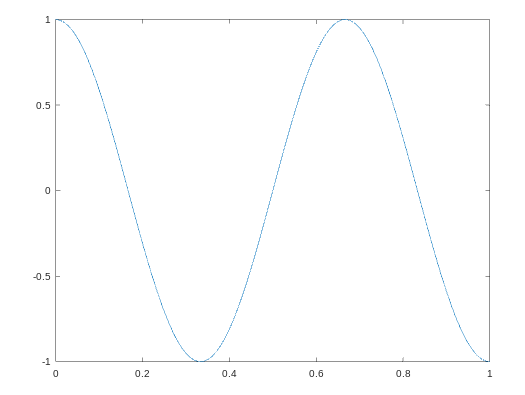Example 3 : We can plot, one plot over another plot by holding the previous plot with the hold on command.

MATLAB

 % declaring variable var_xvar_x = [0:0.01:1]; % declaring variable var_y1var_y1 = sin(4 * pi * var); % declaring variable var_y2var_y2 = cos(3 * pi * var); % plot var_x with var_y1plot(var_x, var_y1); % hold the above plot or figurehold on; % plot var with var_y2 with red colorplot(var_x, var_y2, 'r');

Output :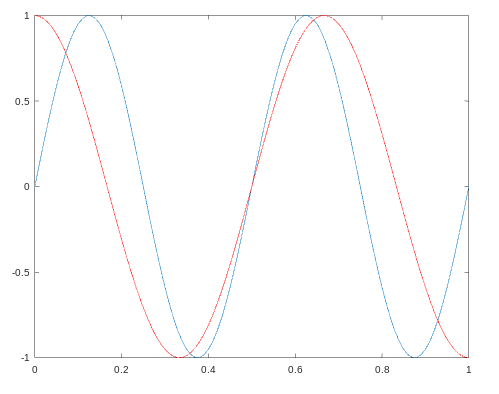Example 4 : We can add labels for the x-axis and the y-axis along with the legends and title with the below code.

MATLAB

 % declaring variable var_xvar_x = [0:0.01:1]; % declaring variable var_y1var_y1 = sin(4 * pi * var);  % declaring variable var_y2var_y2 = cos(3 * pi * var); % plot var_x with var_y1plot(var_x, var_y1); % hold the above plot or figurehold on; % plot var with var_y2 with red colorplot(var_x, var_y2, 'r'); % adding label to the x-axisxlabel('time'); % adding label to the y-axisylabel('value'); % adding title for the plottitle('my first plot'); % add legends for these 2 curveslegend('sin', 'cos');

Output :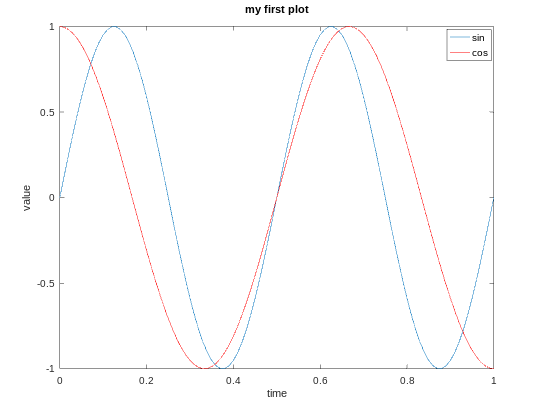Example 5 : We can also plot data on different figures.

MATLAB

 % declaring variable var_xvar_x = [0:0.01:1];  % declaring variable var_y1var_y1 = sin(4 * pi * var);  % declaring variable var_y2var_y2 = cos(3 * pi * var); % plot var_x and var_y1 on figure 1figure(1);plot(var_x,var_y); % plot var_x and var_y2 on figure 2figure(2);plot(var_x,var_y2);

Output :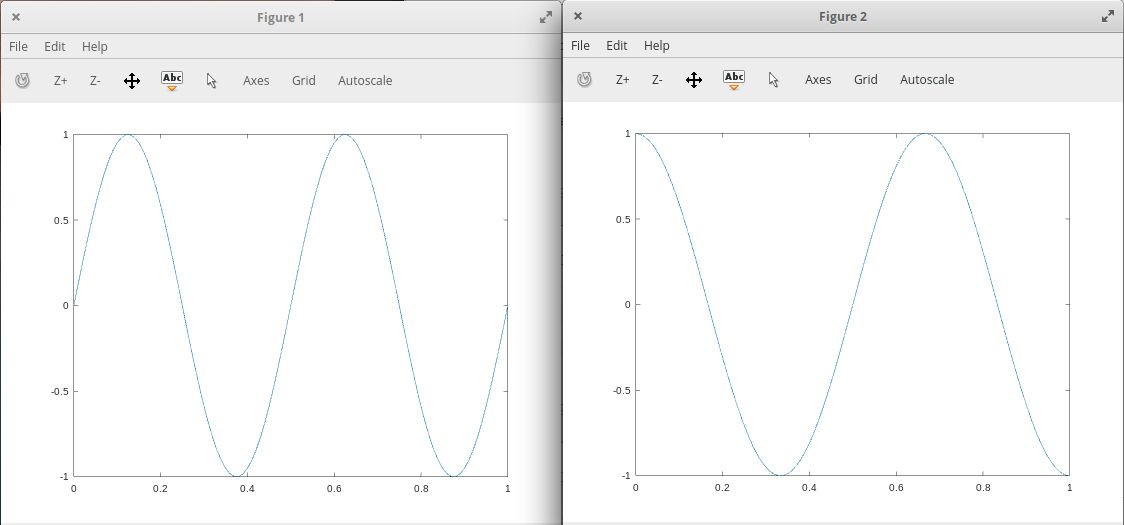Example 6 : We can divide a figure into a m x n grid using the subplot() function. In the below code the first 2 parameter shows m and n and 3rd parameter is the grid count from top to left.

MATLAB

 % var_x for the y-axisvar_x = [0:0.01:1];  % var_y for the y-axisvar_y = sin(4 * pi * var); % plot the var_x and var_y on a 3x3 grid% at 4 position counting from top to leftsubplot(3, 3, 4), plot(var_x, var_y);

Output :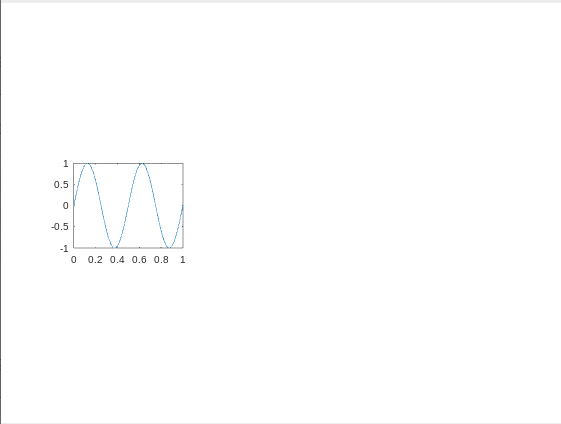Example 7 : We can change the axis values of any plot using the axis() function.

MATLAB

 % declaring variable var_xvar_x = [0:0.01:1];  % declaring variable var_y1var_y1 = sin(4 * pi * var);  % declaring variable var_y2var_y2 = cos(3 * pi * var);  % plot var_x with var_y1plot(var_x, var_y1);  % hold the above plot or figurehold on;  % plot var with var_y2 with red colorplot(var_x, var_y2, 'r'); % adding label to the x-axisxlabel('time'); % adding label to the y-axisylabel('value');  % adding title for the plottitle('my first plot');  % add legends for these 2 curveslegend('sin', 'cos'); % first 2 parameter sets the x-axis% and next 2 will set the y-axisaxis([0.5 1 -1 1])

Here the first 2 parameters shows the range of the x-axis and the next 2 parameters shows the range of the y-axis.
Output :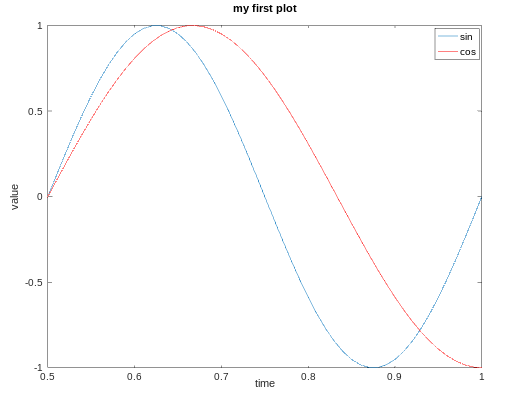Example 8 : We can save our plots in our present working directory :

MATLAB

 print -dpng 'plot.png'

In order to print this plot at our desired location, we can use cd with it as shown below :

MATLAB

 cd '/home/dikshant/Documents'; print -dpng 'plot.png'

We can close a figure/plot using the close command.
Example 9 : We can visualize a matrix using the imagesc() function.

MATLAB

 % creating a 10x10 magic matrixmatrix = magic(10) % plot the matriximagesc(matrix)

Output :

matrix =

92    99     1     8    15    67    74    51    58    40
98    80     7    14    16    73    55    57    64    41
4    81    88    20    22    54    56    63    70    47
85    87    19    21     3    60    62    69    71    28
86    93    25     2     9    61    68    75    52    34
17    24    76    83    90    42    49    26    33    65
23     5    82    89    91    48    30    32    39    66
79     6    13    95    97    29    31    38    45    72
10    12    94    96    78    35    37    44    46    53
11    18   100    77    84    36    43    50    27    59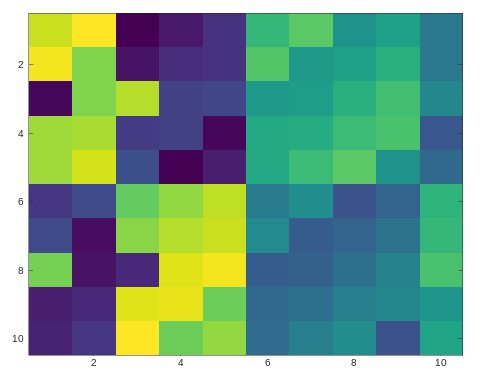The above plot is of 10×10 grid, each grid represents a value with a color. The same color value results in the same color.
We can also make a color bar with this plot to see which value corresponds to which color using the colorbar command. We can use multiple commands at a time by separating them with a comma(,) in Octave environment.

MATLAB

 % creating a 10x10 magic matrixmatrix = magic(10) % plot this matrix with showing colorbar on the right of itimagesc(matrix), colorbar;

Output :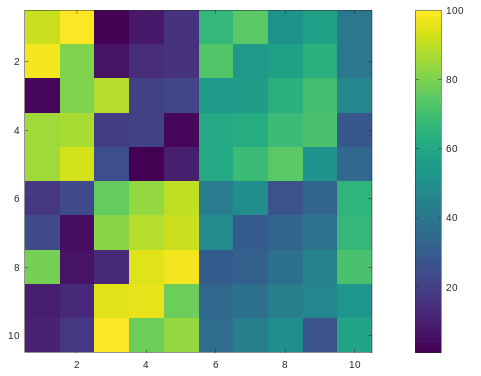Drawing the magic square with a gray-scale colormap :

MATLAB

 % creating a 10x10 magic matrixmatrix = magic(10) % plot this matrix with colorbar and gray colormapimagesc(matrix), colorbar, colormap gray;

Output :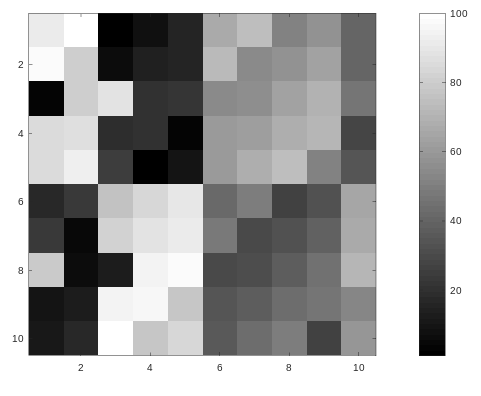My Personal Notes arrow_drop_up